# Hypergeometrical Electromagnetism and Gravitation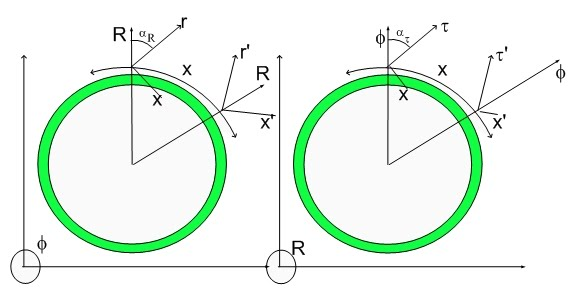Hypergeometrical Electromagnetism and Gravitation

In the past, I corrected the Law of Gravitation. It just happens that when creating the Hypergeometrical Universe paradigm, I had to also create the link from a purely geometric theory into standard physics which has constructs like mass, force, electrostatic permittivity etc. The first thing to do was to recover the Natural Laws discovered experimentally by Newton, Gauss, Biot-Savart, Faraday, etc.

To recover those equations I had to make approximations. Here I present the correct equations for Electromagnetism. They differ from Biot-Savart in a similar manner as my Hypergeometrial Law of Gravitation differs from Newton's Law.

We recovered the Biot-Savart law for infinitesimal elements of current. This was achieved by considering the many contributions of positive and negative center charges and using the low velocity approximation. Within a Tokamak Nuclear Fusion device, currents are both positive and negative (hot plasma) and velocities are relativistic. Under these conditions one should use the non-approximated equation derived from equation (3.41):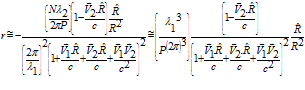The force between two 1 Kg dilators is given by: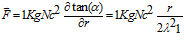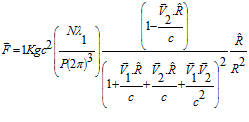To scale this force into the force between two Coulomb charges traveling with velocities v1 and v2 one just have to multiply the equation by (1C/Nqe)2: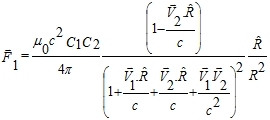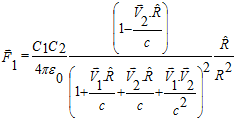if you remember the correct Law of Gravitation from the Gyrogravity posting: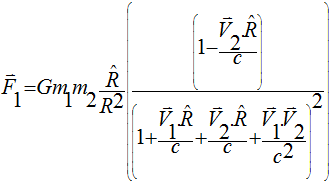Where C1(m1) and C2(m2) are the charges(masses) traveling at V1 and V2 and c is the speed of light. R points from charge 2 to charge 1.

You might have noticed that all forces have basically the same equation. ..J

This is called Grand Unification...:).  It is the first time one has an equation that would work for all forces of nature - according to my theory.

Of course, there have been prior theories in which "Grand Unification" was partially achieved. Kaluza-Klein achived unification of Gravitation and Electromagnetism.  That was done in a contrived non-physical manner.  It introduced an extra column in the metric corresponding to an extra dimension. This dimension, as all the other that came after, was a compact dimension. It was necessary to create electromagnetic modes or other quantum numbers.

Now-a-days, Physics is full of compact dimensions which I deem non-physical.  Every other time a new particle is discovered a new "curled dimension" is proposed.  I don't see any frugality in the creation of dimensions..:) I see dimensional inflation... :)

I mentioned "Grand Unification" because I don't see the need for Strong and Weak Forces in the description of Nature. I, certainly don't see need for none of the many curled dimensions..:) My Hypergeometrical Universe Standard model and the concept of a Fundamental Dilator seems to to be able to explain all Hyperons and their decaying reactions.  It also provides masses to all particles without the need for a God Particle or a Bosonic Field.

I will talk more about masses when I finally managed to explain how it is calculated. Of course, the particles associated with the four phases of the fundamental dilator (proton, electron, positron and antiproton) are self-evident and don't need any explanation.

Please feel free to make velocity zero and recover Gauss' and Newton's Laws or make the appropriate approximations (for small velocity with respect to c) and recover Biot-Savart Law.

Some "scientists" which are really attached to Maxwell's equations - after all one can derive the wave equation out of it and that is neat - will say that Biot-Savart Law is a lesser law.  I disagree. Biot-Savart is a law that in the small velocity regimen describes the interaction between moving charges.  In deriving it, I realized and exposed many of the approximations associated with that Natural Law.

My equations are free of those approximations and fascinatingly have v/c in it.  The derivation did start with a Lorentz transformation..:) How come one obtain a relativitistic equation out of a simple model...:)

With respect to waves, they are just declared as existing as the result of dilaton shape shifting.  One might need to add that space is elastic.

It should be heart breaking to realize that one should set aside certain things up and just keep what is relevant to evolve..:)

Cheers,

MP

Currently unrated

### New Comment

required

required (not published)

optional

required